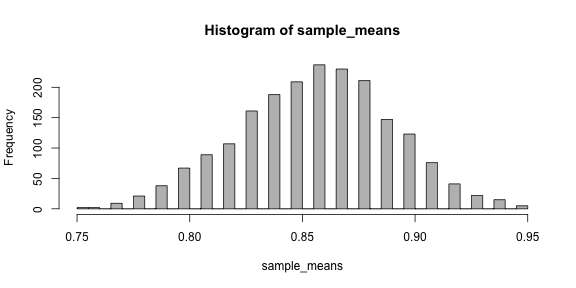Blog of Andrés Aravena
CMB2:

# Homework 10

05 May 2019. Deadline: Friday, 10 May, 9:00.

# Shall you take the umbrella?

It is early in the morning and you are preparing to get out of home. You look out through the window and you see that the sky is cloudy, and it looks like it may rain. You open your cellphone and check the weather prediction. It says 15% probability of rain for each hour on the next 12 hours. So, the chance of rain for each specific hour is small, but you will be out for many hours. Shall you take the umbrella?

We will assume that the probability of rain in every hour is independent of each other. That is, the chance of rain between 8:00 and 9:00 is 15%, and the probability of rain between 9:00 and 10:00 is also 15%.

## 1. Simulate one day

Please write a function called one_day, that simulates one day of possible rain, hour by hour. The function has two inputs: the number of hours h, and the probability of rain for each hour p. The function must return a logic vector of length h. Each element of the vector is TRUE when there is rain on the corresponding hour.

one_day <- function(h, p) {
# write here
}

Notice that the first element of the vector correspond to the time you will leave home, and the last element represents the time just before you come home.

Using the following test code, you should get a plot similar to this figure

hours_of_rain <- replicate(1000, sum(one_day(12, 0.15)))
barplot(table(hours_of_rain)/1000)## 2. How long will it rain

What is the average number of hours of rain? what is the standard deviation?

# write here
 1.883

 1.221804

## 3. Will today be a rainy day?

Please write a function that represents the event “there is rain at least one time”. The function is called rainy_day(v) and takes a vector of logic values, such as the output of the previous question. The output of rainy_day(v) is a single logic value, which is TRUE if there is rain at least for one hour. In other words, rainy_day() is an event.

rainy_day <- function(v) {
# write here
}

Test your function with this code:

rainy_day(c(FALSE, FALSE, FALSE))
 FALSE
rainy_day(c(FALSE, TRUE, FALSE))
 TRUE

## 4. How many rainy days like today?

Write a function that calculates the average number of rainy days. The function must simulate m days and calculate the proportion of days when it rains at least during one hour. Use the functions mean(), replicate(), rainy_day(), and one_day()

mean_rainy_days <- function(m, hours, prob) {
# write here
}

Now you can simulate several samples and see the distribution. Your result should be similar to this:

sample_means <- replicate(2000, mean_rainy_days(100, 12, 0.15))
hist(sample_means, col="grey", nclass=30)## 5. Finding a range for the probability of rain

What is the average of the average number of rainy days? That is, what is your estimation of the population mean?

What is the standard deviation? What is the standard error?

# write here